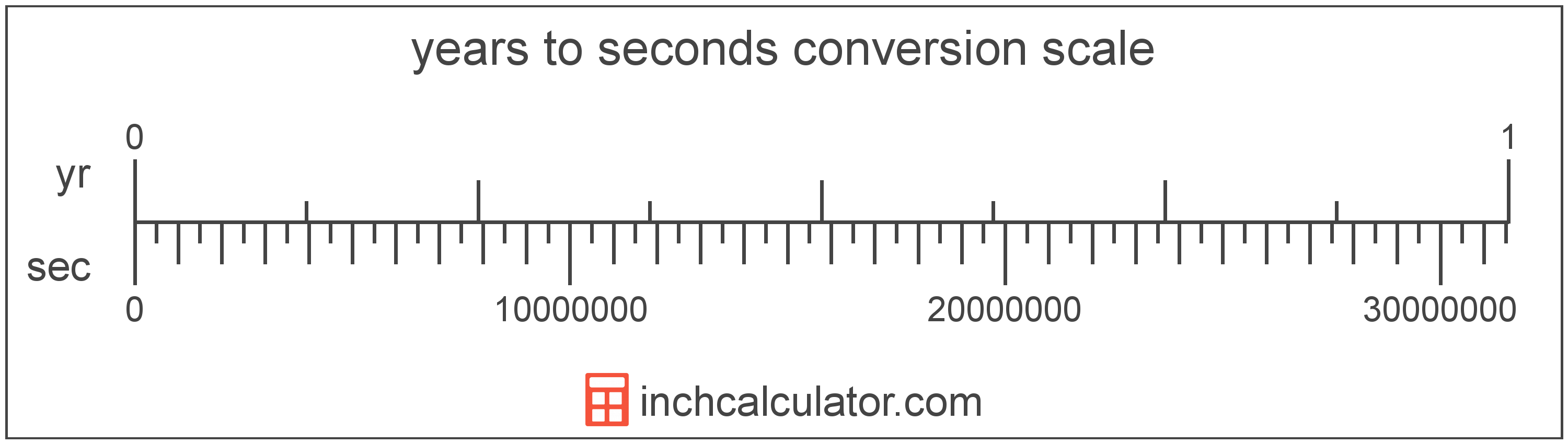# Years to Seconds Converter

Enter the time in years below to get the value converted to seconds.

Results in Seconds:1 yr = 31,556,952 s

Do you want to convert seconds to years?

## How to Convert Years to Seconds

To convert a measurement in years to a measurement in seconds, multiply the time by the following conversion ratio: 31,556,952 seconds/year.

Since one year is equal to 31,556,952 seconds, you can use this simple formula to convert:

seconds = years × 31,556,952

The time in seconds is equal to the time in years multiplied by 31,556,952.

For example, here's how to convert 5 years to seconds using the formula above.
seconds = (5 yr × 31,556,952) = 157,784,760 s### How Many Seconds Are in a Year?

There are 31,556,952 seconds in a year, which is why we use this value in the formula above.

1 yr = 31,556,952 s

## What Is a Year?

One year is the time it takes for the Earth to complete its orbit around the Sun. There are 365 days in a year, with the exception of the leap year when there are 366.

We have leap years because the Earth actually takes 365.2425 days to revolve around the sun, but we round to 365 for convenience. However, since we miss a quarter of a day per revolution around the sun, this means that we add an extra day to the year once every 4 years, hence the leap year.

Years can be abbreviated as yr (plural yrs), and are also sometimes abbreviated as y. For example, 1 year can be written as 1 yr or 1 y, and 2 years can be written as 2 yrs.

## What Is a Second?

The second has historically been defined as 1/60 of a minute or 1/86,400 of a day. According to the SI definition, the second is the duration of 9,192,631,770 oscillations of the frequency of light emitted from the atomic transition between the two hyperfine levels of the unperturbed ground state of the caesium 133 atom.

The second is the SI base unit for time in the metric system. Seconds can be abbreviated as s, and are also sometimes abbreviated as sec. For example, 1 second can be written as 1 s or 1 sec.

## Year to Second Conversion Table

Table showing various year measurements converted to seconds.
Years Seconds
0.0000001 yr 3.1557 s
0.0000002 yr 6.3114 s
0.0000003 yr 9.4671 s
0.0000004 yr 12.62 s
0.0000005 yr 15.78 s
0.0000006 yr 18.93 s
0.0000007 yr 22.09 s
0.0000008 yr 25.25 s
0.0000009 yr 28.4 s
0.00000001 yr 0.31557 s
0.0000001 yr 3.1557 s
0.000001 yr 31.56 s
0.00001 yr 315.57 s
0.0001 yr 3,156 s
0.001 yr 31,557 s
0.01 yr 315,570 s
0.1 yr 3,155,695 s
1 yr 31,556,952 s

## References

1. International Bureau of Weights and Measures, The International System of Units, 9th Edition, 2019, https://www.bipm.org/documents/20126/41483022/SI-Brochure-9-EN.pdf